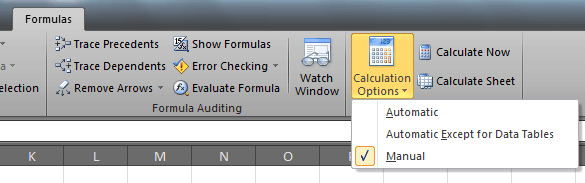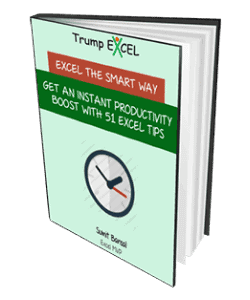# Volatile Formulas Detected in Excel – Keep Your Distance

Last week, I came across an excel problem in a forum. I immediately sprung into action and created a long formula that started with OFFSET().

Within a few hours, it was shot down by other excel experts as it contained volatile formulas.

I immediately recognized the cardinal sin I had committed.

So with this confession, let me share what I have learned about volatile functions in Excel. In plain simple terms, it is a function that will make your excel spreadsheet slow, as it recalculates the formula again and again. A number of actions can trigger this (described later in this post).

A very simple example of a volatile function is the NOW() function (to get the current date and time in a cell). Whenever you edit any cell in a worksheet, it gets recalculate. This is fine if you have a small data set and less number of formulas, but when you have large spreadsheets, this could significantly slow down the processing.

Here is a list of some common volatile functions, which should be avoided:

Super Volatile Formulas:

Almost Volatile Formulas:

The good news is, my favorite INDEX(), ROWS(), and COLUMNS() don’t exhibit volatility. The bad news is that Conditional Formatting is Volatile

Also, ensure that you do not have these functions inside non-volatile functions, such as IF(), LARGE(), SUMIFS(), and COUNTIFS(), as this would eventually make the entire formula volatile.

For example, suppose you have a formula =If(A1>B1, “Trump Excel”,RAND()). Now, if A1 is greater than B1, it returns Trump Excel, but if it is not, then it returns RAND(), which is a volatile function.

###### Triggers that recalculate Volatile Formulas
• Entering new data (if Excel is in Automatic recalculation mode).
• Explicitly instructing Excel to recalculate all or part of a workbook.
• Deleting or inserting a row or column.
• Saving a workbook while the ‘Recalculate before save’ option is set (it’s in File–> Options–> Formula).
• Performing certain Autofilter actions.
• Double-clicking a row or column divider (in Automatic calculation mode).
• Adding, editing, or deleting a defined name.
• Renaming a worksheet.
• Changing the position of a worksheet in relation to other worksheets.
• Hiding or unhiding rows, but not columns.

If you have a lot of formulas in your worksheet that are making it slow, I suggest you switch to Manual Calculation Mode. This stops automatic recalculation and gives you the power to tell excel when to calculate (by clicking ‘Calculate Now’ or pressing F9). This option is available in Formulas–> Calculation Options.###### Related Tutorials:FREE EXCEL BOOK

## Get 51 Excel Tips Ebook to skyrocket your productivity and get work done faster

### 5 thoughts on “Volatile Formulas Detected in Excel – Keep Your Distance”

1. I try to avoid this problem in my Excel environment, but this blog illustrates that “errors” can sometimes be useful when used intelligently. Thank you!

2. I have a very complex workbook that contained multiple NOW() and TODAY() references and as it grew in size through the year the macro that ran transaction posting begin to take longer and longer.

1. I replaced all references to NOW() and TODAY() with a pointer to a cell in my TBL worksheet named NVDate (Non-Volatile Date).

2. Then I added the following code to the ThisWorkbook object:

Private Sub Workbook_Open()
‘Seed NVDate to eliminae volatile Today() and Now() functions
TBL.Range(“NVDate”).Value = Date
End Sub

The gain in speed was astounding. A transaction that had been taking 9 seconds to post was now running in less than a second.

• Thanks for this code. Very helpful, but FOLLOWING DETAIL might be helpful to some.

Private Sub Workbook_Open()
‘Seed NVDate to eliminae volatile Today() and Now() functions
Worksheets(“TBL”).Range(“NVDate”).Value = Date
End Sub

3. vlookup() is volatile as well afaik

4. Nice